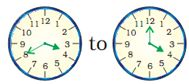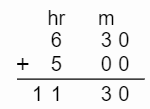### Time and calender - Worksheets

CBSE Worksheet-01

Class - IV Mathematics – Time and calender

Time Allowed: 30 minutes

1. Fill in the blanks with suitable words:
1. In a leap year, February month has ______ days.

2. 1:15 p.m is_____ hour and _____ minutes ahead of 10:45 a.m.

3. 1000 years = _______ centuries.

4. 9 min =______ seconds.

5. The hour hand takes _____ hours to move from 5 to 6 on the clock.

2. State whether each of the following statement is True or False:
1. Period of 10 years makes a century.

2. January month has 30 days.

3. In 24 hours clock system, 11 noon is denoted as 11:00 hours.

4. We can write "twenty to 8" for 7:40.

5. 6.45 p.m. in a 24-hour clock system is 18:45 hours.

3. If Lalit takes 19 hours and 20 minutes and reaches home at 8:30 a.m., then at what time did he start?

1. It is 8:30 p.m.

2. It is 1:50 p.m.

3. It is 1:10 p.m.

4. It is 7:20 p.m.

4. A tap was opened into an empty tank at 9:20 a.m. The tank was full at 10:15 a.m. How long did it take to fill the empty tank?

1. 1 hour

2. 50 minutes

3. 45 minutes

4. 55 minutes

5. 20:20 hrs is ________ in the 12 hour clock time.

1. 08:20 pm

2. 10:20 pm

3. 10:20 am

4. 8:20 am

6. How long will it take the minutes hand to move from:1. 20 minutes

2. 25 minutes

3. 10 minutes

4. 15 minutes

7. Reena left her house for school at 9:05 a.m. and reached school at 9:45 a.m. How much time did she take to reach school from her house?

1. 40 minutes

2. 50 minutes

3. 35 minutes

4. 45 minutes

8. Match the following:

 (a)  43200 seconds (I)  30 minutes (b)  60 seconds (ii) 1 hour (c)  3600 seconds (iii)  12 hours (d)   1800 seconds (iv)  1 minute
9. How many days will be there in the year 2020? It that a leap year?

10. How many days are from

1. 3rd May to 1st July
2. 5th March to 12th April
11. How many minutes pass between:

1. Half past 8 and quarter past 9
2. Half past 4 and quarter to 5
12. The dance party started at 12:50 and ended at 4:35. How long did the dance party take?

13. A bus leaves Ahmedabad for Bhavnagar at 6:30 a.m. It takes 5 hours to reach Bhavnagar, at what time it will reach Bhavnagar. After reaching Bhavnagar, it takes another 2:30 hours to reach the exact bus stop along with the traffic. Find the final time at which bus reaches the final bus stop.

14. Convert the following time into a 12-hour clock system.

1. 20:45
2. 14:42
3. 06:23
4. 04:20
15. Convert the following time into a 24-hour clock system.

1. 3:15 a.m.
2. 9:35 a.m.
3. 2:15 p.m.
4. 6:30 p.m.
16. APRIL 2020

 Monday Tuesday Wednesday Thursday Friday Saturday Sunday 1 2 3 4 5 6 7 8 9 10 11 12 13 14 15 16 17 18 19 20 21 22 23 24 25 26 27 28 29 30

Answer the following questions using the above calendar of April 2020.

1. which is the first day of the month
2. How many times Tuesday come in April 2020?
3. which is the last day of the month?
4. find the date on the second Sunday?

CBSE Worksheet-01
Class - IV Mathematics – Time and calender

Solution

1. Fill in the blanks with suitable words:

1. 29. In a leap year, February month has 29 days.

2. 2 hours and 30 minutes.
10:45 a.m to 11:45 a.m = 1 hours.
11:45 a.m to 12:45 p.m = 1 hours.
12:45 p.m to 1:15 p.m = 30 minutes.

3. 10
100 years = 1 century.
1000 years = $\frac{1000}{100}$century = 10 centuries

4. 540.
As we know, 1 min = 60 seconds
Then, 9 min = 9$×$60 = 540 seconds

5. one. The hour hand takes 1 hour to move from 5 to 6 on the clock.

2. State whether each of the following statement is True or False:

1. FALSE
Period of 100 years makes a century.

2. False
January month has 31 days.

3. True
In 24 hours clock system, 11 noon is denoted as 11:00 hours.

4. True, We can write "twenty to 8" for 7:40.

5. True
6.45 p.m. in a 24-hour clock system is 18:45 hours.
6.45 p.m. = 12:00 + 06: 45 = 18:45 hours

3. (c) It is 1:10 p.m.
Explanation: Lalit takes 19 hours and 20 minutes and reaches home at 8:30 a.m.
From 12 to 8.30 a.m. 8.30 hours
So 19.20 – 8.30 =  10 hours 50 minutes
10 hours 50 minutes before 12 means 1:10 p.m.
So Lalit started at 1:10 p.m.
4. (d) 55 minutes
Explanation: A tap was opened into an empty tank at 9:20 a.m.
Tank was full at 10:15 a.m.
Difference = 10: 15 – 9: 20 = 55 minutes
So 55 minutes required to fill the empty tank.
5. (a) 08:20 pm
Explanation: 20:20 hrs is represent after 12 pm so, it represent as 08:20 in the 12 hour clock time.
6. (a) 20 minutes
Explanation: First clock show: 3:40 and second clock show: 4:00
So difference = 20 minutes
7. (a) 40 minutes
Explanation: Reena left her house for school at 9:05 a.m. and reached school at 9:45 a.m.
Time she take to reach school from her house = 9:45 a.m. – 9:05 am = 40 minutes
8. (a) – (iii), (b) – (iv), (c) – (ii), (d) – (i)

• 1 minutes = 60 seconds
• 30 minutes = 30 $×$ 60 =1800 seconds
• 1 hours = 60 $×$ 60 = 3600 minutes
• 12 hours = 3600 $×$ 12 = 43200
9. There 366 days in year 2020. Yes, it is the leap year 2020.

1. 3rd May to 1st July
Days from 3rd May to 31 May = 29 days
Days in June month = 30 days
So, there are 29 + 30 = 59 days
2. 5th March to 12th April
Days from 5th March to 31st March = 27 days
Day from 1st April to 12th April = 11 days
So, there are 27 + 11 = 38 days
1. Half past 8 and quarter past 9
We can write Half past 8 as 8:30 and quarter pass 9 as 9:15
Minute pass between 8:30 to 9:00 = 30 minutes
Minute pass between 9:00 to 9:15 = 15 minutes
So, the minute pass between 8:30 to 9:15 is (30+15) = 45 minutes.
2. Half past 4 and quarter to 5
We can write Half past 4 as 4:30 and quarter to 5 as 4:45
Minute pass between 4:30 to 4:45 = 15 minutes
So, the minute pass between 4:30 to 4:45 is 15 minutes.
10. The dance party started at 12:50 and ended at 4:35.
$12:50\phantom{\rule{thickmathspace}{0ex}}\stackrel{10\phantom{\rule{thickmathspace}{0ex}}\mathrm{m}\mathrm{i}\mathrm{n}\mathrm{u}\mathrm{t}\mathrm{e}\mathrm{s}}{\to }\phantom{\rule{thickmathspace}{0ex}}1:00\phantom{\rule{thickmathspace}{0ex}}\stackrel{3\phantom{\rule{thickmathspace}{0ex}}\mathrm{h}\mathrm{o}\mathrm{u}\mathrm{r}}{\to }\phantom{\rule{thickmathspace}{0ex}}4:00\stackrel{35\phantom{\rule{thickmathspace}{0ex}}\mathrm{m}\mathrm{i}\mathrm{n}\mathrm{u}\mathrm{t}\mathrm{e}\mathrm{s}\phantom{\rule{thickmathspace}{0ex}}}{\to }\phantom{\rule{thickmathspace}{0ex}}4:35$
10 minutes + 3 hours + 35 minutes = 3 hours 45 minutes

11. A bus leaves Ahmedabad for Bhavnagar at 6:30 a.m. It takes 5 hours to reach Bhavnagar,Bus reaches at Bhavnagar at 11:30 a.m. and takes 2:30 hours to reach the stop.
So, 11:30 hours + 2:30 hours is 14:00 hours.
Here, 14:00 hours =  2:00 pm.
So, the bus will reach the bus stop at 2:00 pm.

• for A.M. 24 hour clock time and 12 hour clock time both are the same.
• for P.M. to convert 24 hour clock time to 12 hour clock time we have to subtract 12 in 24 hour clock time.
1. 20:45
20:45 - 12:00 = 8:45 p.m.
2. 14:42
14:42 - 12:00 = 2:45 p.m.
3. 06:23
$⇒$ 06:23 a.m.
4. 04:20
$⇒$ 04:20 a.m.
• for A.M. 24 hour clock time and 12 hour clock time both are the same.
• for P.M. to convert  12 hour clock time to 24 hour clock time we have to add 12 in 12hour clock time.
1. 3:15 a.m.
3:15 a.m. $⇒$ 03:15
2. 9.35 a.m.
9:35 a.m. $⇒$ 09:35
3. 2:15 p.m.
2:15 p.m. $⇒$ 12:00 + 2:15 = 14:15
4. 6:30 p.m.
6:30 p.m. $⇒$ 12:00 + 6:30 = 18:30
1. Wednesday
2. 4 times
3. Thursday
4. 12/04/2020